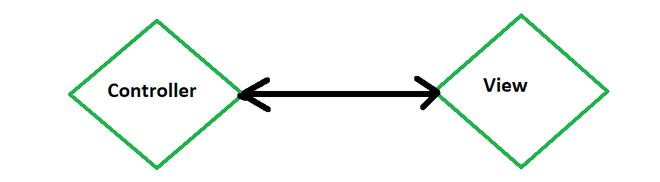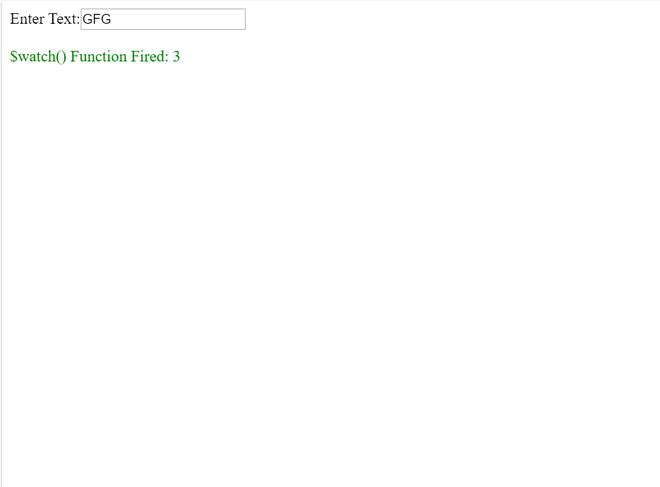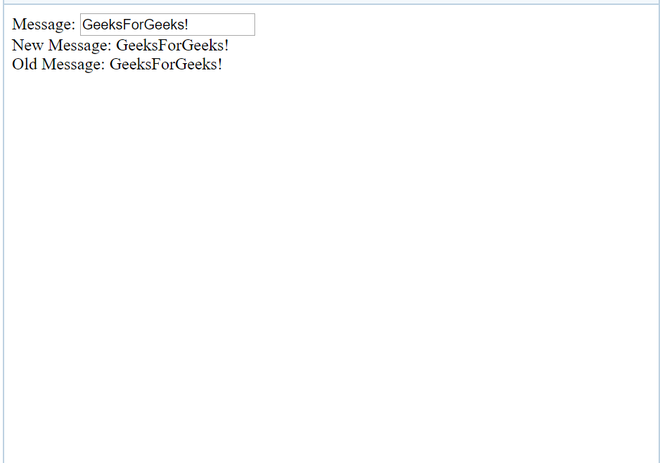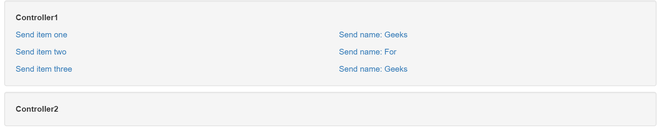# How to watch service variables ?

• Last Updated : 14 Jul, 2022

\$scope acts as a built-in object in AngularJs.

It consists of application data and methods. The \$scope is acts as a link between a controller and view (HTML).\$scope is used to transfer the data.

\$watch:

Scope in AngularJs object has \$watch event which comes into picture when the model property gets changed or altered.

When a data binding is created from some point of program in the view to a variable on the \$scope object, a watch is created internally in AngularJs.

The digest gets called multiple times, when we \$watch() a function.

Every time when we bind the UI, We insert \$watch in \$watch list.

```User: <input type="text" ng-model="user" />

Example:

## html

 ```<``html``>``    ``<``head``>``        ``<``title``>``            ``AngularJs \$watch() Function with GFG``        ````        ``<``script` `src=``"https://ajax.googleapis.com/ajax/libs/angularjs/1.4.8/angular.min.js">``      ````        ``<``script` `type="text/javascript">``            ``var app = angular.module("watchApp", []);``            ``app.controller("watchCtrl", function (\$scope) {``                ``\$scope.count = -1;``                ``\$scope.\$watch(``                  ``"txtval", function (newval, oldval) {``                    ``\$scope.count = \$scope.count + 1;``                ``});``            ``});``        ````    ````    ``<``body``>``        ``<``div` `ng-app="watchApp"``             ``ng-controller="watchCtrl">``            ``Enter Text:<``input` `type="text"``                              ``ng-model="txtval" />``          ``<``br` `/>``            ``<``br` `/>``            ``<``span` `style="color: Green;">``              ``\$watch() Function Fired: {{count}}``        ````    `````Output

\$watch in AngularJs is a service.

It is used to keep the track of the changes on specific properties within a given scope. It is similar to an event listener. e changes are made in the value of specified variables.

When we register a watch we pass two functions as parameters to the \$watch() function.These functions are:

• A value function
• A listener function

Example:

```\$scope.\$watch(function() {}, //value function
function() {}  //listener function
);```

A watcher can change in responses on:

1. Timeouts
2. UI
3. Complex asynchronous computations performed by web workers
4. Ajax calls

Methods Used: \$scope.\$watchGroup

\$watchGroup() is a shortcut to set up watchers with the same callback, passing an array of watchExpressions.

\$scope.\$watchGroup([‘obj.a’, ‘obj.b.bb’, ‘letters’], function(newValues, oldValues, scope) { //… });

\$scope.\$watchCollection is a shortcut to watch arrays or objects. In arrays, the listener is called when any of the elements are replaced, deleted, or added.

The \$watch keeps track of the variable. This function takes two arguments:

new value

\$scope.\$watch(‘expression’, function (newvalue, oldvalue) { //expression parameter //Code });

old value

\$scope.\$watch(function () {}, function (newvalue, oldvalue) { //Function Parameter // Code });

Example:

## html

 ```<``html``>``<``head``>``    ``<``script` `src=``"https://ajax.googleapis.com/ajax/libs/angularjs/1.3.16/angular.min.js">``   ``````<``body` `ng-app="myNgApp">` `    ``<``div` `ng-controller="myController">``        ``Message: <``input` `type="text"``                        ``ng-model="message" />``        ``<``br` `/>``        ``New Message: {{newMessage}} <``br` `/>``        ``Old Message: {{oldMessage}}``    ````    ``<``script``>``        ``var ngApp = angular.module('myNgApp', []);``    ` `        ``ngApp.controller('myController', function (\$scope) {``            ``\$scope.message = "GeeksForGeeks!";` `            ``\$scope.\$watch(``'message', function (newValue, oldValue) {``                ``\$scope.newMessage = newValue;``                ``\$scope.oldMessage = oldValue;``            ``});``        ``});``    ```````Output

Others:

\$digest()

We call the \$digest() function when AngularJS thinks it is necessary.

For example, after a button click  handler is executed, or after an AJAX call returns.

\$apply():

The \$scope.\$apply() function takes a function as parameter which it is executed, and after that \$scope.\$digest() is called internally. That makes it helpful to all the watches are checked

```\$scope.\$apply(function() {
\$scope.data.myVar = "value";
});```

Example of \$Watch:

## html

 `<``div` `class="container" data-ng-app="app">``    ``<``div` `class="well" data-ng-controller="FooCtrl">``        ``<``p``><``strong``>Controller1``        ``<``div` `class="row">``            ``<``div` `class="col-sm-6">``                ``<``p``><``a` `href=""``                      ``ng-click=``   ``"setItems([ { name: 'I am single item' } ])">``                  ``Send item one ``                ``<``p``><``a` `href=""``                      ``ng-click=``               ``"setItems([ { name: 'Item 1 of 2' },``                  ``{ name: 'Item 2 of 2' } ])">``                  ``Send item two ``              ` `                ``<``p``><``a` `href=""``                      ``ng-click=``                      ``"setItems([ { name: 'Item 1 of 3' },``                  ``{ name: 'Item 2 of 3' },``                  ``{ name: 'Item 3 of 3' } ])">``                  ``Send item three ``            ````            ``<``div` `class="col-sm-6">``                ``<``p``><``a` `href=""``                      ``ng-click="setName('Sheldon')">``                  ``Send name: Geeks``                ``<``p``><``a` `href=""``                      ``ng-click="setName('Leonard')">``                  ``Send name: For``                ``<``p``><``a` `href=""``                      ``ng-click="setName('Penny')">``                  ``Send name: Geeks``            ````        ````    ``` `    ``<``div` `class="well"``         ``data-ng-controller="BarCtrl">``        ``<``p``><``strong``>Controller2``        ``<``p` `ng-if="name">Name is: {{ name }}``        ``<``div` `ng-repeat="item in items">``          ``{{ item.name }}``    `````

## javascript

 `var` `app = angular.module(``'app'``, []);` `app.factory(``'PostmanService'``, ``function``() {``  ``var` `Postman = {};``  ``Postman.set = ``function``(key, val) {``    ``Postman[key] = val;``  ``};``  ``Postman.get = ``function``(key) {``    ``return` `Postman[key];``  ``};``  ``Postman.watch = ``function``(\$scope, key, onChange) {``    ``return` `\$scope.\$watch(``      ` `      ``function``() {``        ``return` `Postman.get(key);``      ``},``      ` `      ``function``(newValue, oldValue) {``        ``if` `(newValue !== oldValue) {``          ``// Only update if the value changed``          ``\$scope[key] = newValue;``          ``// Run onChange if it is function``          ``if` `(angular.isFunction(onChange)) {``            ``onChange(newValue, oldValue);``          ``}``        ``}``      ``}``    ``);``  ``};``  ``return` `Postman;``});` `app.controller(``'FooCtrl'``, [``'\$scope'``,``                           ``'PostmanService'``,``             ``function``(\$scope, PostmanService) {``  ``\$scope.setItems = ``function``(items) {``    ``PostmanService.set(``'items'``, items);``  ``};``  ``\$scope.setName = ``function``(name) {``    ``PostmanService.set(``'name'``, name);``  ``};``}]);` `app.controller(``'BarCtrl'``, [``'\$scope'``,``                           ``'PostmanService'``,``           ``function``(\$scope, PostmanService) {``  ``\$scope.items = [];``  ``\$scope.name = ``''``;``  ``PostmanService.watch(\$scope, ``'items'``);``  ``PostmanService.watch(``       ``\$scope,``'name'``, ``function``(newVal, oldVal) {``    ``alert(``'Hi, '` `+ newVal + ``'!'``);``  ``});``}]);`

CSS:

## css

 `.well {`` ``margin-top``: ``10px``;`` ``margin-bottom``: ``10px``;``}` `p:last-child {`` ``margin-bottom``: ``0``;``}`Output

My Personal Notes arrow_drop_up